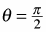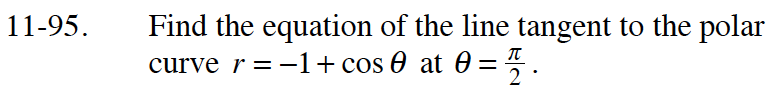### Home > CALC > Chapter 11 > Lesson 11.3.1 > Problem11-95

11-95.

Find the equation of the line tangent to the polar curve r = − 1 + cos θ at. Homework Help ✎To write the equation of the line, the slope, or dy/dx is needed.

For the given value of θ, where is the point on the curve?
What are the coordinates of this point in rectangular form?

$x=r\cos(\theta)=(-1+\cos(\theta))(\cos(\theta))$

$y=r\sin(\theta)=(-1+\cos(\theta))(\sin(\theta))$

Write an equation for:

Evaluate your slope equation from Step 3 using the (rectangular) point (0, –1).